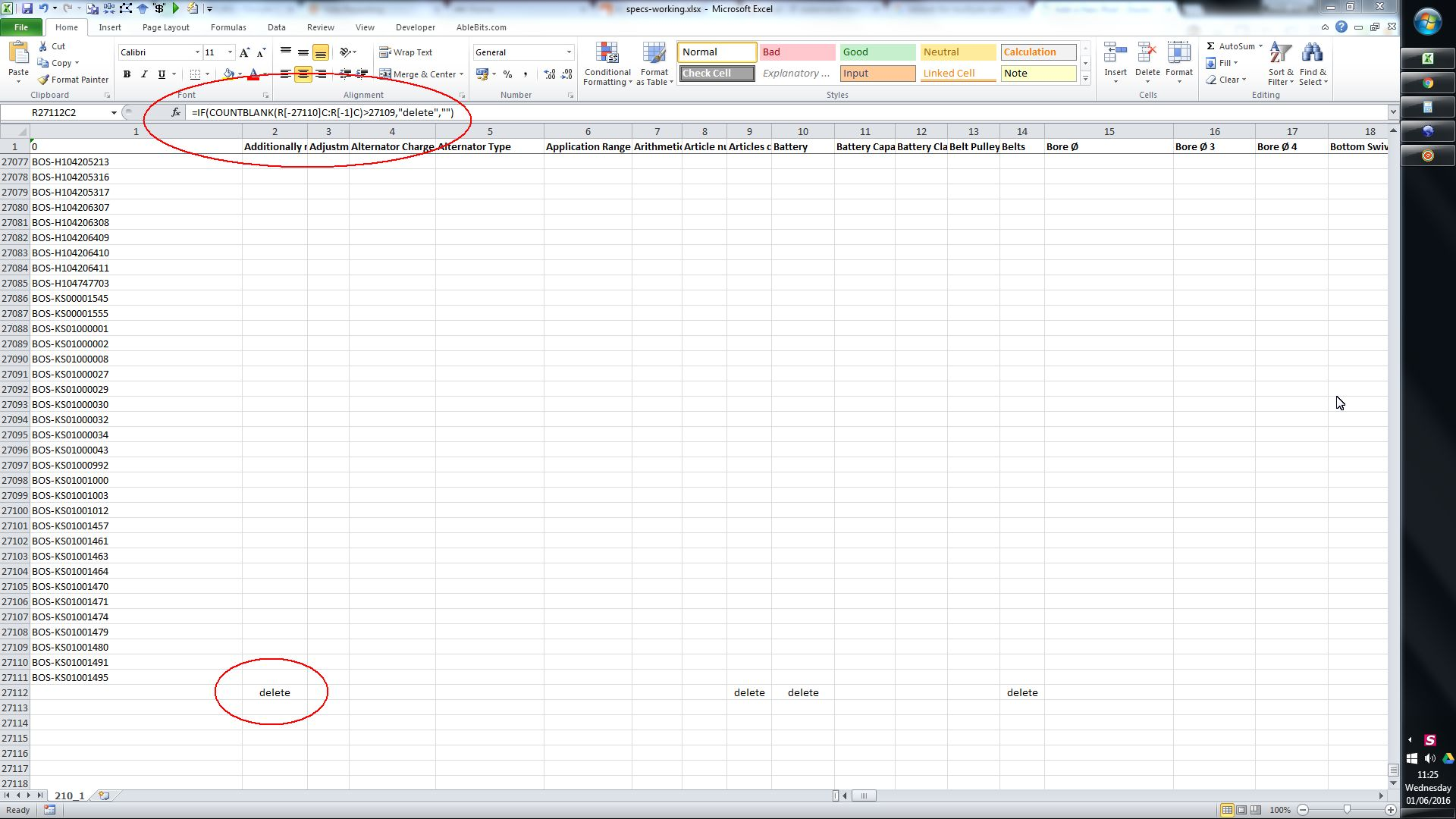# How to Delete Empty Columns in Excel with all Empty / Blank Cell Values

On the net you will find lots of info on how to delete empty columns in excel, using ‘go to special’. These are useless if you have some columns which have ‘some blanks’ and are looking for columns which have ‘only blanks’, because the method will delete the entire column even if it contains one blank cell.

I prefer instead to use the COUNTBLANK formula. Simply go to the last row/column of the data, and use countblank to find how many cells are empty/blank. then combine it with an IF statement, so that if the number of blanks is greater than the total number of columns/rows, then mark ‘delete’, and if not leave blank.

After this you simply sort your data by the column/row where you did the formula, and delete the relevant columns/rows. (Remember, when trying to delete empty columns, you will need to sort the data by the row which contains the formula. You do this by opening the ‘sort’ box and then pressing ‘options’ button)

Formula:

IF(COUNTBLANK(R[-27110]C:R[-1]C)>27109,”delete”,””)

in the above example, 27109 was the number of rows of data in my data – yours will be a different number.